Chapter 4 Class 11 Mathematical Induction
Concept wise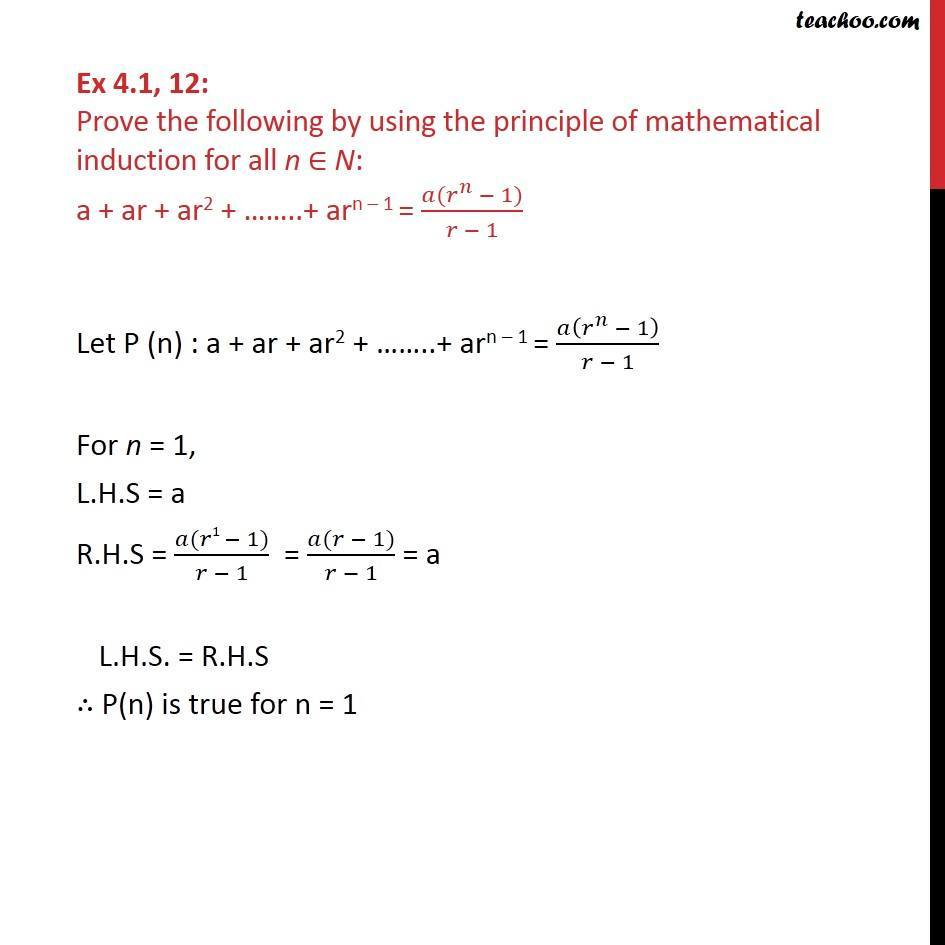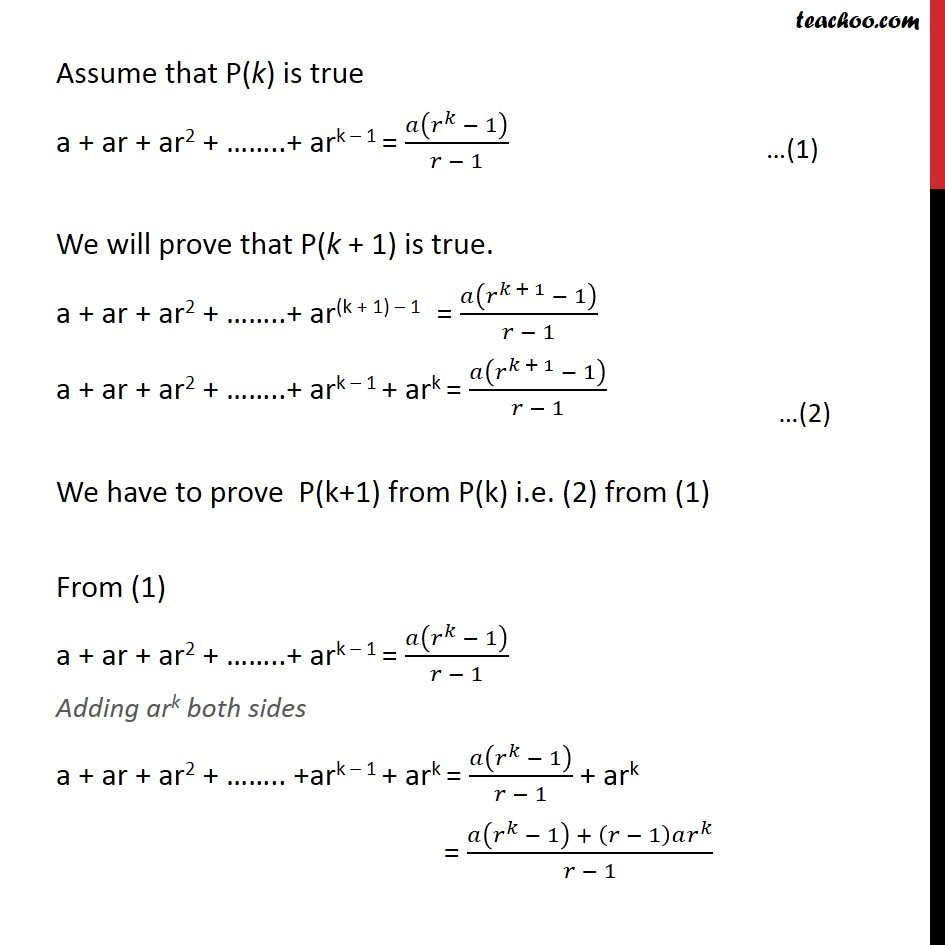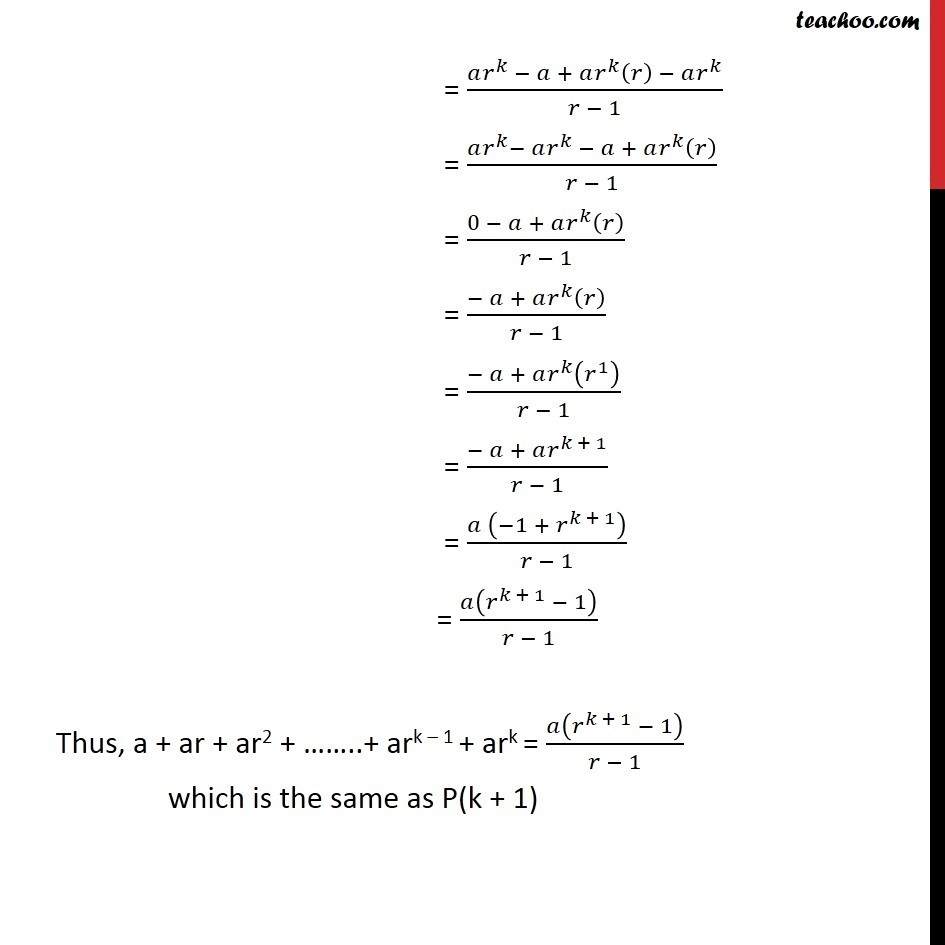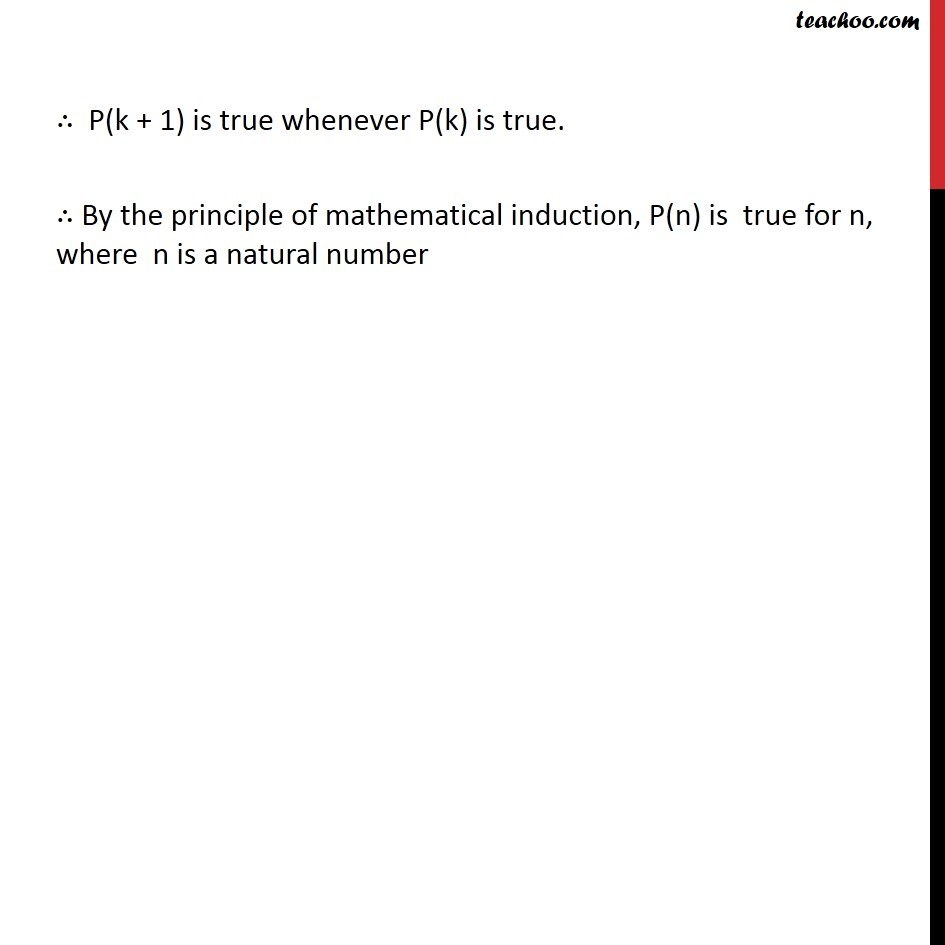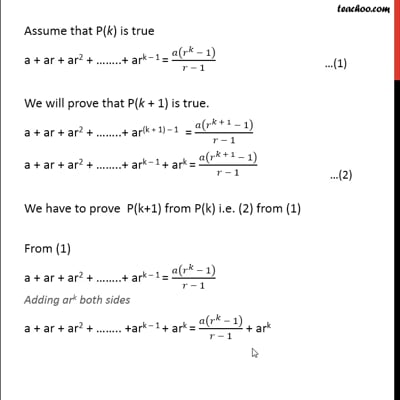This video is only available for Teachoo black users

Introducing your new favourite teacher - Teachoo Black, at only ₹83 per month

### Transcript

Ex 4.1, 12: Prove the following by using the principle of mathematical induction for all n ∈ N: a + ar + ar2 + ……..+ arn – 1 = (𝑎(𝑟^𝑛 − 1))/(𝑟 − 1) Let P (n) : a + ar + ar2 + ……..+ arn – 1 = 𝑎(𝑟^𝑛 − 1)/(𝑟 − 1) For n = 1, L.H.S = a R.H.S = (𝑎(𝑟1 − 1))/(𝑟 − 1) = (𝑎(𝑟 − 1))/(𝑟 − 1) = a L.H.S. = R.H.S ∴ P(n) is true for n = 1 Assume that P(k) is true a + ar + ar2 + ……..+ ark – 1 = 𝑎(𝑟^𝑘 − 1)/(𝑟 − 1) We will prove that P(k + 1) is true. a + ar + ar2 + ……..+ ar(k + 1) – 1 = 𝑎(𝑟^(𝑘 + 1) − 1)/(𝑟 − 1) a + ar + ar2 + ……..+ ark – 1 + ark = 𝑎(𝑟^(𝑘 + 1) − 1)/(𝑟 − 1) We have to prove P(k+1) from P(k) i.e. (2) from (1) From (1) a + ar + ar2 + ……..+ ark – 1 = 𝑎(𝑟^𝑘 − 1)/(𝑟 − 1) Adding ark both sides a + ar + ar2 + …….. +ark – 1 + ark = 𝑎(𝑟^𝑘 − 1)/(𝑟 − 1) + ark = (𝑎(𝑟^𝑘 − 1) + (𝑟 − 1)𝑎𝑟^𝑘)/(𝑟 − 1) = (𝑎𝑟^𝑘 − 𝑎 + 𝑎𝑟^𝑘 (𝑟) − 𝑎𝑟^𝑘)/(𝑟 − 1) = (𝑎𝑟^𝑘− 𝑎𝑟^𝑘 − 𝑎 + 𝑎𝑟^𝑘 (𝑟))/(𝑟 − 1) = (0 − 𝑎 + 𝑎𝑟^𝑘 (𝑟))/(𝑟 − 1) = (− 𝑎 + 𝑎𝑟^𝑘 (𝑟))/(𝑟 − 1) = (− 𝑎 + 𝑎𝑟^𝑘 (𝑟^1 ))/(𝑟 − 1) = (− 𝑎 + 𝑎𝑟^(𝑘 + 1))/(𝑟 − 1) = (𝑎 (−1 + 𝑟^(𝑘 + 1) ))/(𝑟 − 1) = 𝑎(𝑟^(𝑘 + 1) − 1)/(𝑟 − 1) Thus, a + ar + ar2 + ……..+ ark – 1 + ark = 𝑎(𝑟^(𝑘 + 1) − 1)/(𝑟 − 1) which is the same as P(k + 1) ∴ P(k + 1) is true whenever P(k) is true. ∴ By the principle of mathematical induction, P(n) is true for n, where n is a natural number